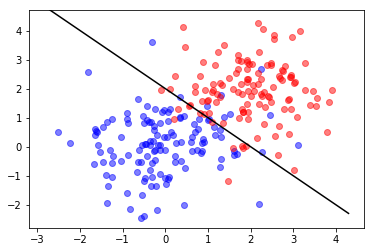In :
%matplotlib inline
import matplotlib.pyplot as plt
import numpy as np
import scipy as sp
import scipy.stats as st
import scipy.linalg as la
from math import sqrt
print("Modules Imported!")

Modules Imported!

In :
# plot helper function for plotting a dataset along with the boundaries of classifiers
def classPlot(thetas):
# thetas = list of vectors theta, where each theta has three elements
colors = ['k','g','m','y']
fig, ax = plt.subplots()
ax.scatter(X0[:,0],X0[:,1],color='b',alpha=.5) # X0: x-coordinates for class 0 datapoints
ax.scatter(X1[:,0],X1[:,1],color='r',alpha=.5) # X1: x-coordinates for class 1 datapoints
ylim = ax.get_ylim()
x0_range = np.array(ax.get_xlim())
for i in range(len(thetas)):
theta = thetas[i]
x1_range = [(-theta - theta*a)/theta for a in x0_range]
ax.plot(x0_range,x1_range,colors[i])
ax.set_ylim(ylim);


## Binary Classification with Gaussian Class-Conditionals¶

(25 pts) Build and plot a classiffier assuming that the parameters of the generative model are known. First, we will generate the data for each class by drawing random samples from a multivariate normal distribution using the following parameters:

m0 = [0,0] # mean of class 0
m1 = [2,2] # mean of class 1
K = [[1,0],[0,1]] # Covariance matrix for both classes
N = 250 # number of data points
pi = 1/2 # the probability that a data point belongs to class 1

Next, assuming that the parameters for the generative model are known, we build a binary classifier using Gaussian Class-Conditionals. To be consistent with following problems, formulate your classifier as:

$$\theta_0 x_0 + \theta_1 x_1 + \theta_2 \gtrless 0.$$

We then plot the data points and the line indicating the decision boundary in one plot, using the plot helper function classPlot defined above. The input for this function is a list of vectors representing classifiers, each of the form theta=[$\theta_0$,$\theta_1$,$\theta_2$].

In :
m0 = np.array([0,0])
m1 = np.array([2,2])
K = np.array([[1,0],[0,1]])

N = 250
pi = 1/2
N1 = st.binom.rvs(N,pi)
N0 = N - N1
X0 = ...
X1 = ...
...## Maximum Likelihood¶

(25 pts)Similar to the previous part, we are going to build a binary classifier over the same dataset but this time we will be using ML to estimate the parameters of the generative model from the data. i.e. the values of pi, m0, m1 and sigma are all computed from the data.

we then plot the data points and the ML decision line along with the true decision line (obtained in the previous part) in one plot, using the same plot helper function classPlot.

In :
# solution


## Gradient Descent for Logistic Regression¶

(25 pts) Let us assume a logistic regression model. First, we modify the existing data points from the previous parts by concatanting a 1 to each data point (so each data point is of the form x = [x0,x1,1]).

We then use gradient descent to find the optimized values for theta with 1000 iterations with a step size equal to $0.001$ to build the classifier.

Similar to the previous part we plot this classifier generated using Gradient descent along with the other two classifiers in a single plot.

In [ ]:
# solution


## Stochastic Gradient Descent for Logistic Regression¶

(25 pts) In this part, we replace the Gardient Descent from the previous part with a Stochastic Gradient Descent using a step size of $t^{-3/4}$ and 1000 iterations to obtain the optimised value of theta. Plot the classifier so obtained along with the previously computed classfiers using the helper function classPlot.

In [ ]:
# solution